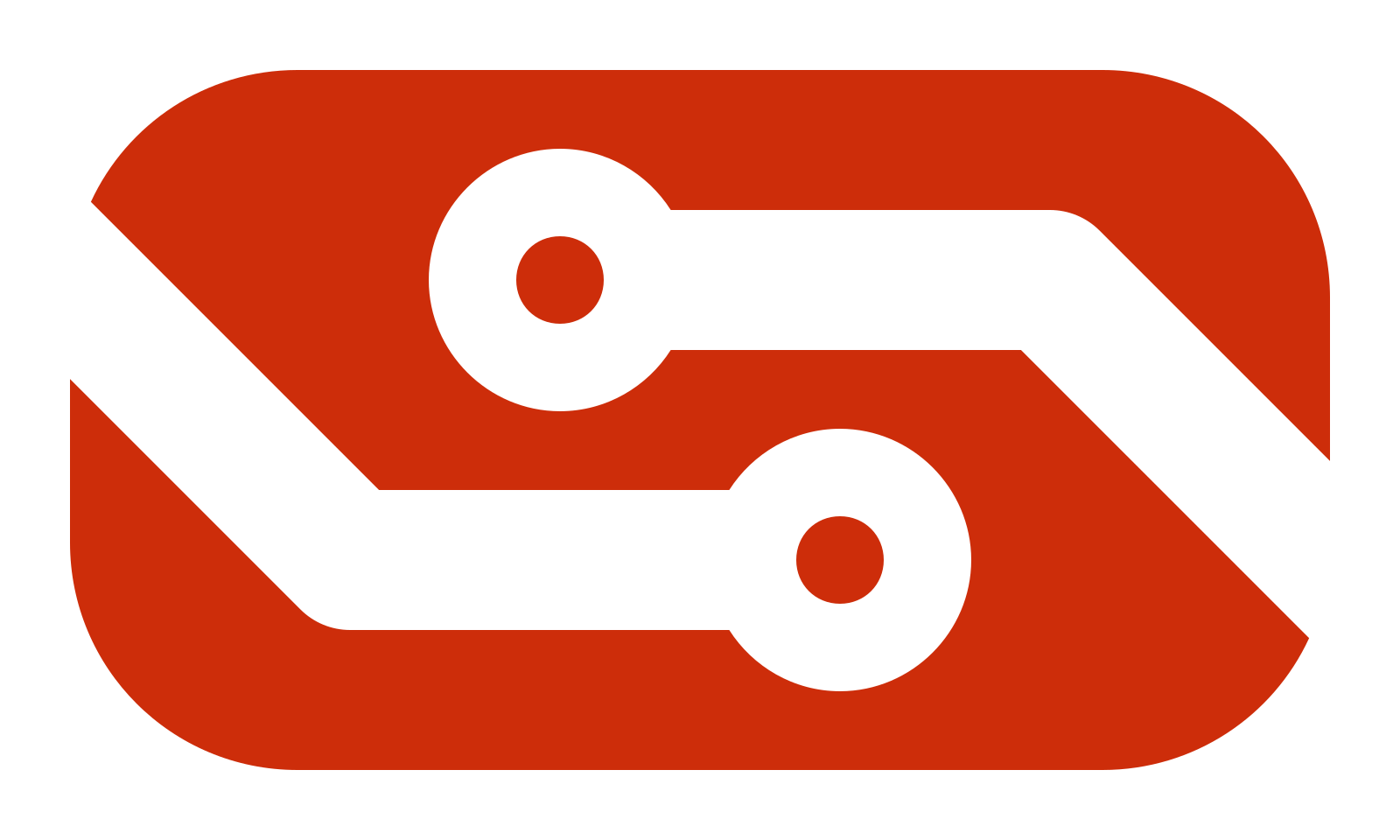Binary

Index

An index of all Computational Redstone Guides by IAmLesbian, this one included.

None

Binary

Binary is a number system, similar and different to the one we use. But, before we dive head-first into a new number system, let’s take a minute to look at the number system we already use.

Decimal

The number system you are most familiar with is likely Decimal, or Base Ten. We count from 0 to 9 with a single digit, then we run out of digits and go up to 10. Each digit is worth ten times as much as the digit to its right. So 100 is ten times more than 10. Additionally, if we add together two digits and they are more than ten, we carry a 1.

3 + 8 = 11

Hopefully this is all nothing new, just a review of facts we aren’t actively thinking about. Now with that fresh in our minds, we can move into Binary.

Binary

Binary, or Base Two, works similar to Decimal. The main difference is that instead of having ten digits, Binary only has two; 0 and 1. In Binary, digits are normally called “bits”. Counting in Binary starts just like it does in Decimal.

0, 1…

But then we run out of digits, and need to carry over.

0, 1, 10, 11…

And again

0, 1, 10, 11, 100, 101, 110, 111…

Binary, having only two values, doesn’t increase by ten times each bit, but rather by two times. So 100 is two times as much as 10.

You might be thinking: “How can I tell if a number is in Binary or in Decimal?” The best way to distinguish between the two is by using special prefixes. Often, when Binary numbers are used, they will be prefixed with 0b to help avoid confusion. The rest of this guide will use that prefix.

Binary to Decimal Conversion

Now that we’ve covered the basics of counting in Binary, let’s get into some conversion. Counting from 0x0 up to a given Binary number works, but eventually the numbers get too big for it to be practical. Let’s start with a simple example and work our way up.

Say we wanted to convert 0b101 into Decimal. How would we go about that? Well, we want to figure out the place values and sum up any with a 1. Here’s that done for our example.

Place Values   4 | 2 | 1
----------
Bits           1 | 0 | 1
----------
Sum            4 + 0 + 1 = 5

So 0b101 = 5, simple enough. But what about a larger value like 0b10001011? Let’s find out

Place Values   128 | 64 | 32 | 16 | 8 | 4 | 2 | 1
-----------------------------------
Bits           1   | 0  | 0  | 0  | 1 | 0 | 1 | 1
-----------------------------------
Sum            128 + 0  + 0  + 0  + 8 + 0 + 2 + 1 = 139

0b10001011 = 139, cool. So that is how you convert a Binary number to Decimal. But what about the other way around?

Decimal to Binary Conversion

Converting from Decimal is a different process than the other way around. This way is more iterative. Let’s start with 19.

19 >= 16 (subtract)
3 = 19 - 16

Our first step says 19 is greater than or equal to 16. After that, we subtract the 16 from 19. The idea here is that we want to take the largest place value we can without going lower than 0. We want to hit 0, not miss it. 32 is larger than 19, so we start with 16.

3 < 8 (skip)
3 = 3 - 0

Next, we have 8. 8 is larger than 3, so we skip 8 and don’t subtract anything.

3 < 4 (skip)
3 = 3 - 0

Here is the same, 4 is larger than 3. Skip 4.

3 >= 2 (subtract)
1 = 3 - 2

Now we have 3 greater than or equal to 2. Which means we can subtract.

1 >= 1 (subtract)
0 = 1 - 1

Lastly, we have 1. Our value is exactly 1, so we subtract it and hit 0. This is where we stop.

19 = 16 + 2 + 1

So now we have our number in terms of Binary place values.

Place Values  16 | 8 | 4 | 2 | 1
-------------------
Bits          1  | 0 | 0 | 1 | 1 = 0b10010

So now all we have left is to turn those place values into a binary number. And now we have our result. 19 = 0b10010.

Continue

Currently, there is no guide to follow this one. Once there is, this will be updated to include a link to it.

1 Like